# SSAT Middle Level Math : How to find mode

## Example Questions

← Previous 1

### Example Question #1 : How To Find Mode

Give the mode of the following data set: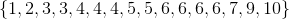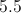The set has four modes: 3, 4, 5, and 6.

The set has no mode.Explanation:

The element 6 appears four times, more frequently than any other element, and is therefore the (only) mode.

### Example Question #2 : How To Find Mode

Find the mode in this set:

856, 479, 256, 245, 256, 659, 213, 256, 478, 789.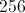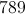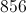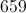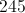Explanation:

Identify the number that appears most frequently.

The answer is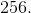### Example Question #1 : How To Find Mode

Find the mode in this set: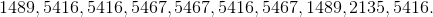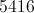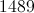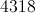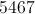Explanation:

Fing the number that appears most frequently in the set.

The answer is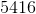.

### Example Question #4 : How To Find Mode

Find the mode of this set of numbers: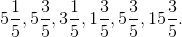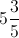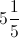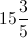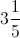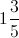Explanation:

Find the number that appears most frequently.

The answer is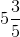.

### Example Question #5 : How To Find Mode

What is the mode for the following set of numbers?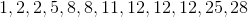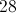Explanation:

The mode is the number that occurs most often in the list.  Since 12 appears three times, and no other number shows up as often, the mode is 12.

### Example Question #6 : How To Find Mode

John loves to play video games, and in a new game, his point scores have been 12, 12, 11, 11, 12, 10, and 9 for the 7 games that he has played so far. What is the mode of his scores?Explanation:

A mode is a number (or score in this case) that occurs the most in a set. In this set, the number 12 occurs three times, the most of any of the numbers. Therefore, it is the mode and the correct answer is 12.

### Example Question #7 : How To Find Mode

What is the mode of this set of numbers?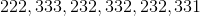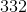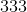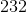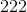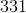Explanation:

In a set, the mode is the number that appears most frequently.The number 232 is the number that appears most frequently because is the only number that appears twice. Therefore, it is the mode.

### Example Question #8 : How To Find Mode

Over the past five days, John has run the following number of miles: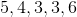If M is equal to the mode, R is equal to the median, and J is equal to the mean, what is the order of M, R, and J from smallest to largest?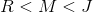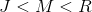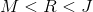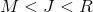Explanation:

The first step is to reorder the setin order from smallest to largest.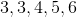The mode (which occurs most often) is 3, so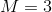The median (which is the middle number in the set) is, so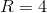The mean (or average) is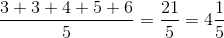,

so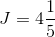Therefore,### Example Question #5 : How To Find Mode

Bruce sells cookies to his neighbors. On Monday, he soldcookies, on Tuesday he soldcookes, on Wednesday he soldcookies, on Thursday he soldcookies, and on Friday he soldcookies. What is the mode of the daily cookies he sold?cookiescookiescookiescookiescookiescookies

Explanation:

The mode is the number that appears the most in a sequence. Given that the numberappears twice while the other numbers only appear once, it is the mode.

### Example Question #514 : Isee Middle Level (Grades 7 8) Mathematics Achievement

Jenny was selling magazines for a fundraiser. Below is the number of magzines she sold per day over the lastdays: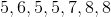What is the mode of the number of magazines that she sold per day?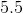Given thatappears more than any other number (times), it is the mode of the set.